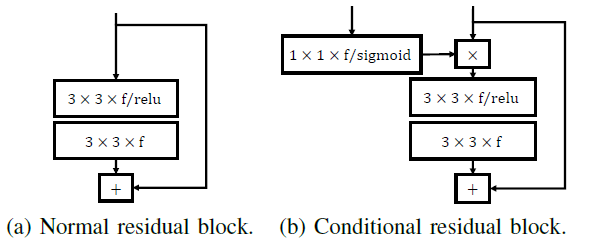Review — Improving Compression Artifact Reduction via End-to-End Learning of Side Information (JPEG Filtering)

Using Artifact Descriptors as Additional Side Information, Outperforms ARCNN, DnCNN, MemNet, EDSR-baseline*.

this story, Improving Compression Artifact Reduction via End-to-End Learning of Side Information, Ma VCIP’20, by University of Science and Technology of China, is reviewed. In this paper:

• The side information consists of artifact descriptors that are obtained by analyzing the original and compressed images in the encoder.
• In the decoder, the received descriptors are used as additional input to a well-designed conditional post-processing neural network.

This is a paper in 2020 VCIP. (Sik-Ho Tsang @ Medium)

Outline

1. Overall Scheme
2. Artifact Descriptor Extraction
3. Quantization
4. Rate Estimation
5. Artifact Feature Mapping
6. Conditional Residual Block
7. Experimental Results

1. Overall Scheme

• Encoder: In the conditional post-processing pipeline, the floating-point artifact descriptors are first obtained by analysing the original and compressed images, with a neural network.
• These descriptors will be quantized and encoded to bitstream as side information.
• Decoder: the received bitstream will be decompressed to reconstruct the descriptors, and then will be mapped to artifact features, which will be used as conditions by the conditional post-processing neural network, with another neural network.
• The training loss similar to  is used:
• where D is the mean square error (MSE) between the outputs and the original images, and R measures the bit rate of the side information.

2. Artifact Descriptor Extraction

• A layer with ”2↓” represents the convolution layer with stride of 2.
• The original image and the compressed image respectively pass through three convolutional layers to extract features fori and frec.
• fori, frec and their differences are then stacked together and input to the subsequent layers.
• A 1×1 convolution and softmax operation are used in the final step to map features to floating-point probability vectors, named as artifact descriptors.
• The channel dimension of the artifact descriptors is set as 16.

3. Quantization

• KL distance is used as it is more suitable for measuring the distance between two probability distributions.
• Specifically, every artifact descriptor x R¹⁶ extracted from an image needs to be quantized to one of the 16 one-hot vectors C = {c1, c2, …, c16} ∈ B¹⁶.
• In the forward pass, x is quantized as the nearest one-hot vector:
• In backward pass, the above equation is approximated by the soft quantization to ensure the back propagation:
• where σ = 1 in this paper.

4. Rate Estimation

• The idea of the context model in  is adopted to accurately estimate the bit rate of the transmitted side information.
• Specifically, one 5 × 5 masked convolution layer and three 1 × 1 convolutional layers are used to implement the context model.
• The outputs have 16 channels and are normalized along channel direction by softmax to obtain the probability vectors pR¹⁶.
• The bit rate can be calculated as follows in the forward pass:
• where X is the set of all quantized artifact descriptors of one image.
• ˆx · pˆx represents vector dot product between vector ˆx and pˆx, and ||X|| is the size of X.

5. Artifact Feature Mapping

• “2↑” and “8↑” represent 2× and 8× up-sampling.
• The convolutional layers identified by “2↑” are implemented by sub-pixel convolutional layers ESPCN .
• The output artifact features have the same resolution as the compressed images.

6. Conditional Residual BlockThe normal residual block suggested in ResNet, and conditional residual block in this paper
• The up-sampling layer of EDSR-baseline neural network in EDSR  is removed, which has 16 residual blocks, and use it as the post-processing neural network backbone, and called EDSR-baseline*.
• For the conditional form, the artifact features are directly multiplied to the input features of residual blocks after a 1 × 1 convolutional layers.

7. Experimental Results

7.1. Complexity Analysis

• From the above table, it can be found that artifact descriptor extracting, artifact feature mapping, and the introduced conditional mechanism only bring small storage and computation overheads.
• The method that training multiple models and choosing in encoder, is also compared.
• In the encoder, the proposed method causes much smaller storage and computation overheads.
• In the decoder, by increasing the computation complexity slightly, the proposed method decreases the storage space significantly.

7.2. Rate Distortion Performance

• A simplified baseline model is also tried, named S-EDSR, by reducing the number of residual blocks in EDSR-baseline* from 16 to 4.
• Accordingly, the two test conditional models are named as EDSR-baseline*+side and S-EDSR+side.
• On LIVE1 dataset, the EDSR-baseline* outperforms ARCNN , DnCNN , and MemNet.
• On DIV2K validation dataset, when the quality factor of JPEG is set to 10, the PSNR of output images of S-EDSR and EDSR-baseline* are both increased by more than 0.9 dB, by using side information.
• When considering the transmission overhead, it can be found that the RD performance is also improved due to the contribution of side information. For example, on DIV2K validation dataset, the S-EDSR+side (with 4 residual blocks) is even comparable with EDSR-baseline* (with 16 residual blocks).